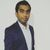# QlikView App Dev

Discussion Board for collaboration related to QlikView App Development.

Announcements
Action-Packed Learning Awaits! QlikWorld 2023. April 17 - 20 in Las Vegas: REGISTER NOW
cancel
Showing results for
Did you mean:Contributor III

## no calculation for the last 7 days

Hello all,

I want to hide the values from the last 7 days in my chart for the Pageviews/ Quantity expression.

i tried with a simple if condition and also with set analysis, but it's not working.

sum({< Date={"<=\$(Date(max(Date)-7,'MM/DD/YYYY'))"} >} Pageviews)

/

sum({< Date={"<=\$(Date(max(Date)-7,'MM/DD/YYYY'))"} >} Quantity)

Regards,

Andrei

1 Solution

Accepted SolutionsMVP

Sum({<Date *= {"<=\$(=Date(max(Date)-7,'MM/DD/YYYY'))"}>} Pageviews)

/

Sum({<Date *= {"<=\$(=Date(max(Date)-7,'MM/DD/YYYY'))"}>} Quantity)

8 RepliesMaster

Try this

sum({< Date={"<=\$(=Date(max(Date)-7,'MM/DD/YYYY'))"} >} Pageviews)

/

sum({< Date={"<=\$(=Date(max(Date)-7,'MM/DD/YYYY'))"} >} Quantity)Contributor III
Author

Partially resolved.

it works, but now, the chart does not considerate the date selection.

for example, when i select april 2018, in the chart all dates will be shown, from 1 jan 2017.

I want to view only april and without last 7 days.

Thx,

A.Master

try this

sum({< Month = {"\$=(Date(max(Date),'MM'))"}, Date={"<=\$(=Date(max(Date)-7,'MM/DD/YYYY'))"} >} Pageviews)

/

sum({< Month = {"\$=(Date(max(Date),'MM'))"}, Date={"<=\$(=Date(max(Date)-7,'MM/DD/YYYY'))"} >} Quantity)

Also let me know if you have month field or not..and what value are you getting in  =(Date(max(Date),'MM'))Contributor III
Author

i don't have a month field.

If i need, i can create one in my script, maybe Year-month is better, because i have April 2017 and April 2018.

the value obtained is 04Contributor III
Author

also, i want to have the option to select 2 months, not only one and to have in my chart only data from those 2 months.

i think the expression with Month will not help meMVP

Sum({<Date *= {"<=\$(=Date(max(Date)-7,'MM/DD/YYYY'))"}>} Pageviews)

/

Sum({<Date *= {"<=\$(=Date(max(Date)-7,'MM/DD/YYYY'))"}>} Quantity)Contributor III
Author

it worked.

wow, that * is magicalThanks a lot!MVP